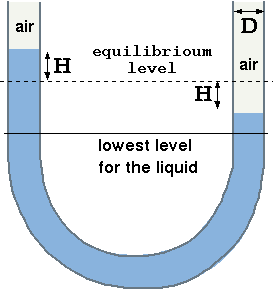# 7.2: Limitation of Integral Approach

$$\newcommand{\vecs}{\overset { \rightharpoonup} {\mathbf{#1}} }$$ $$\newcommand{\vecd}{\overset{-\!-\!\rightharpoonup}{\vphantom{a}\smash {#1}}}$$$$\newcommand{\id}{\mathrm{id}}$$ $$\newcommand{\Span}{\mathrm{span}}$$ $$\newcommand{\kernel}{\mathrm{null}\,}$$ $$\newcommand{\range}{\mathrm{range}\,}$$ $$\newcommand{\RealPart}{\mathrm{Re}}$$ $$\newcommand{\ImaginaryPart}{\mathrm{Im}}$$ $$\newcommand{\Argument}{\mathrm{Arg}}$$ $$\newcommand{\norm}{\| #1 \|}$$ $$\newcommand{\inner}{\langle #1, #2 \rangle}$$ $$\newcommand{\Span}{\mathrm{span}}$$ $$\newcommand{\id}{\mathrm{id}}$$ $$\newcommand{\Span}{\mathrm{span}}$$ $$\newcommand{\kernel}{\mathrm{null}\,}$$ $$\newcommand{\range}{\mathrm{range}\,}$$ $$\newcommand{\RealPart}{\mathrm{Re}}$$ $$\newcommand{\ImaginaryPart}{\mathrm{Im}}$$ $$\newcommand{\Argument}{\mathrm{Arg}}$$ $$\newcommand{\norm}{\| #1 \|}$$ $$\newcommand{\inner}{\langle #1, #2 \rangle}$$ $$\newcommand{\Span}{\mathrm{span}}$$$$\newcommand{\AA}{\unicode[.8,0]{x212B}}$$

Some of accuracy issues to enhance the quality and improvements of the integral method were suggested in the analysis of the emptying tank. There are problems that the integral methods even with these enhancements simply cannot tackle. The improvements to the integral methods are the corrections to the estimates of the energy or other quantities in the conservation equations. In the calculations of the exit velocity of a tank, two such corrections were presented. The first type is the prediction of the velocities profile (or the concentration profile). The second type of corrections is the understanding that averaged of the total field is different from the averaged of different zooms. In the case of the tank, the averaged velocity in $$x$$ direction is zero yet the averaged velocity in the two zooms (two halves) is not zero. In fact, the averaged energy in the $$x$$ direction contributes or effects the energy equation. The accuracy issues that integral methods intrinsically suffers from no ability to exact flow field and thus lost the accuracy as was discussed in the example. The integral method does not handle the problems such as the free surface with reasonable accuracy. Furthermore, the knowledge of whether the flow is laminar or turbulent (later on this issue) has to come from different techniques. Hence the prediction can skew the actual predictions.Fig. 7.5 Flow in an oscillating manometer.

In the analysis of the tank it was assumed that the dissipation can be ignored. In cases that dissipation play major role, the integral does not provide a sufficient tool to analyze the issue at hand. For example, the analysis of the oscillating manometer cannot be carried by the integral methods. A liquid in manometer is disturbed from a rest by a distance of $$H_0$$. The description $$H(t)$$ as a function of time requires exact knowledge of the velocity field. Additionally, the integral methods is too crude to handle issues of free interface. These problem were minor for the emptying the tank but for the oscillating manometer it is the core of the problem. Hence different techniques are required. The discussion on the limitations was not provided to discard usage of this method but rather to provide a guidance of use with caution. The integral method is a powerful and yet simple method but has has to be used with the limitations of the method in mind.